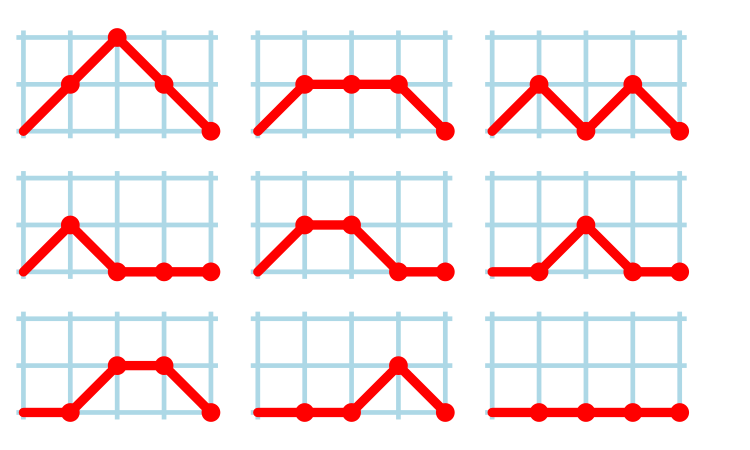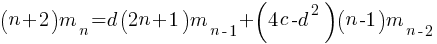## Motzkin paths and source code silhouettes

Consider a language that just contains assignments and if-statements (no `else` arm). Nesting level could be used to visualize programs written in such a language; an `if` represented by an Up step, an `assignment` by a Level step, and the if-terminator (e.g., the `}` token) by a Down step. Silhouettes for the nine possible four line programs are shown in the figure below (image courtesy of Wikipedia). I use the term silhouette because the obvious terms (e.g., path and trace) have other common usage meanings.How many silhouettes are possible, for a function containingstatements? Motzkin numbers provide the answer; the number of silhouettes for functions containing from zero to 20 statements is: 1, 1, 2, 4, 9, 21, 51, 127, 323, 835, 2188, 5798, 15511, 41835, 113634, 310572, 853467, 2356779, 6536382, 18199284, 50852019. The recurrence relation for Motzkin numbers is (whereis the total number of steps, i.e., statements):Human written code contains recurring patterns; the probability of encountering an if-statement, when reading code, is around 17% (at least for the C source of some desktop applications). What does an upward probability of 17% do to the Motzkin recurrence relation? For many years I have been keeping my eyes open for possible answers (solving the number theory involved is well above my mathematics pay grade). A few days ago I discovered weighted Motzkin paths.

A weighted Motzkin path is one where the Up, Level and Down steps each have distinct weights. The recurrence relationship for weighted Motzkin paths is expressed in terms of number of colored steps, where:is the number of possible colors for the Level steps, andis the number of possible colors for Down steps; Up steps are assumed to have a single color:setting:and(i.e., all kinds of step have one color) recovers the original relation.

The different colored Level steps might be interpreted as different kinds of non-nesting statement sequences, and the different colored Down steps might be interpreted as different ways of decreasing nesting by one (e.g., a `goto` statement).

The connection between weighted Motzkin paths and probability is that the colors can be treated as weights that add up to 1. Searching on “weighted Motzkin” returns the kind of information I had been looking for; it seems that researchers in other fields had already discovered weighted Motzkin paths, and produced some interesting results.

If an automatic source code generator outputs the start of an `if` statement (i.e., an Up step) with probability, an assignment (i.e., a Level step) with probability, and terminates the `if` (i.e., a Down step) with probability, what is the probability that the function will contain at leaststatements? The answer, courtesy of applying Motzkin paths in research into clone cell distributions, is:where:is the‘th Catalan number, and that `[...]` is a truncation; code for an implementation in R.

In human written code we know that, because the number of statements in a compound-statement roughly has an exponential distribution (at least in C).

There has been some work looking at the number of peaks in a Motzkin path, with one formula for the total number of peaks in all Motzkin paths of length n. A formula for the number of paths of length, havingpeaks, would be interesting.

Motzkin numbers have been extended to what is called higher-rank, where Up steps and Level steps can be greater than one. There are statements that can reduce nesting level by more than one, e.g., `break`ing out of loops, but no constructs increase nesting by more than one (that I can think of). Perhaps the rather complicated relationship can be adapted to greater Down steps.

Other kinds of statements can increase nesting level, e.g., for-statements and while-statements. I have not yet spotted any papers dealing with the case where an Up step eventually has a corresponding Down step at the appropriate nesting level (needed to handle different kinds of nest-creating constructs). Pointers welcome. A related problem is handling if-statements containing `else` arms, here there is an associated increase in nesting.

What characteristics does human written code have that results in it having particular kinds of silhouettes? I have been thinking about this for a while, but have no good answers.

If you spot any Motzkin related papers that you think could be applied to source code analysis, please let me know.